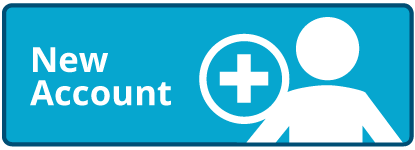# Rounding to the Nearest Ten (Up to 99)

Times up!
Times up! You may finish answering the current question and then proceed to your score!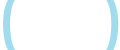Sorry, you have used all of your available hints for this lesson.
Practice Limit Reached

You've reached your daily practice limit of 12 questions.

Award InformationAwarded toQuestion Hint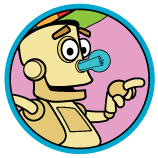Choose Family Member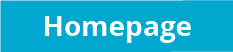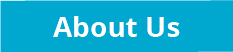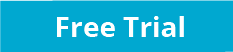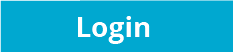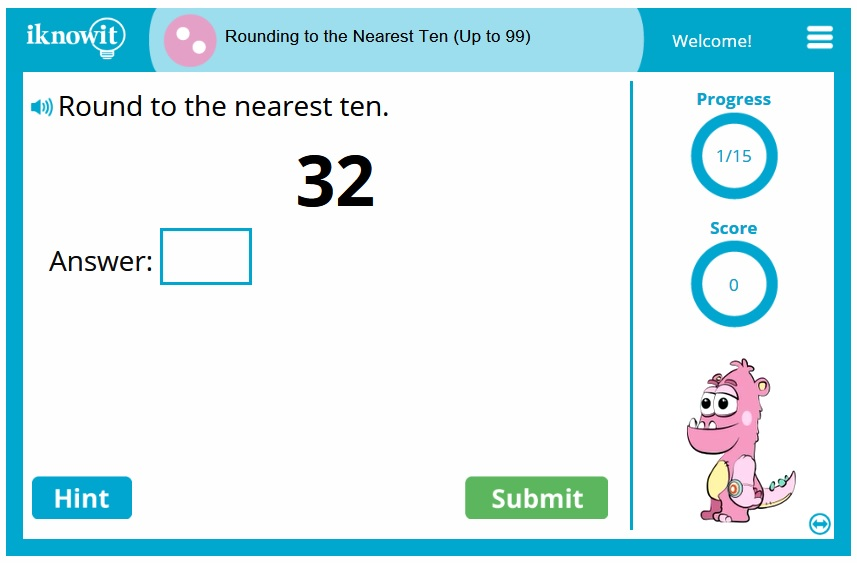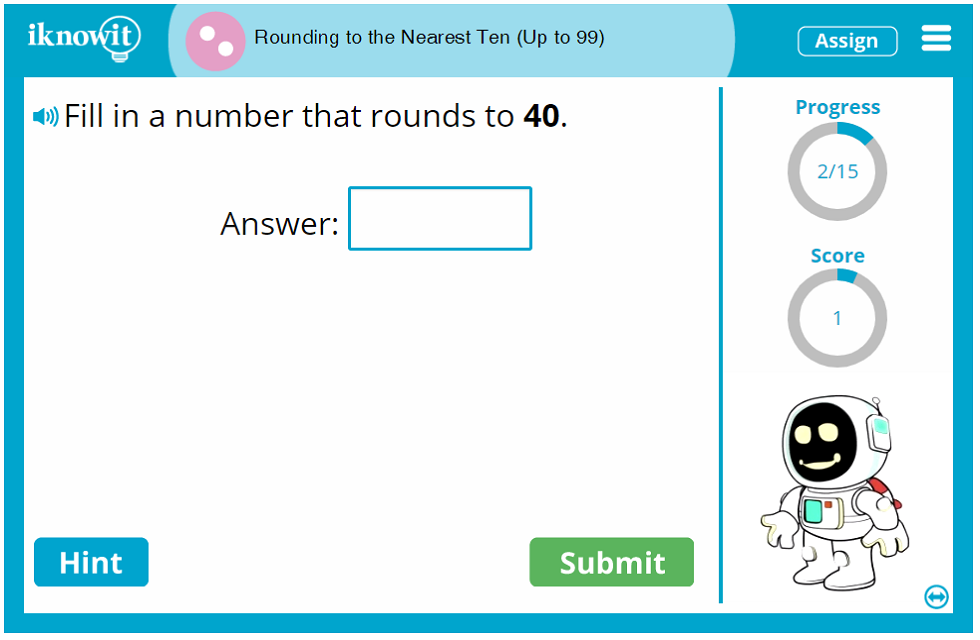## Math Activity: Round to the Nearest Ten (Up to 99)

This interactive math lesson gives students the opportunity to practice rounding numbers to the nearest ten. The students will round numbers up to 99. Several question formats are presented in this entertaining, yet educational, math game. One math question asks students to drag and drop numbers into the correct column to show which numbers round to 70 or 80, for example. In another type of question, students need to fill in a number that rounds to the nearest ten for the given number. Another question format will help kids with rounding to the nearest ten by asking which multiple choice answer rounds to the number given. There are also word problems in this lesson that ask students to round to the nearest ten for a given number.

Students are given a limited amount of hints in this rounding math activity. The hints remind students to look at the ones place. If the number is five or more, round up. If the number is less than five, round down. When a student has used up all his or her hints, the hint window will display this in a message.

Perhaps one of the most invaluable components of this rounding lesson for kids is the detailed explanation that appears when a student gets the answer wrong. Each explanation tells students to look at the ones place to determine whether to round up or round down. If the question is a multiple choice question, the explanation breaks down which answers round up and which ones round down.

When students are immersed this multiplying decimals math activity, they will feel like they are playing a fun, challenging math game. Teachers will see each "I Know It" lesson as an invaluable opportunity for their students to practice basic math skills. It's a win-win for students and teachers alike! You'll love the kid-friendly interface in this interactive math lesson. The questions are displayed in an easy-to-read format. The hint button is just a click away. In the upper-right corner of the screen, a progress icon tells students how many questions they've answered in the lesson. Below the progress icon, a score-keeper lets students know how many points they've accumulated so far as they solve the decimal multiplication questions correctly.

This math activity definitely doesn't feel like math practice when kids are engaged in the rounding to the nearest ten questions! Students think they are playing a fun and challenging math game, while teachers see the benefits of interactive math practice that they can use to monitor their students' progress in rounding to the nearest ten. The interface is very user-friendly and tailored to kids. The hint button in the lower-left corner of the screen is easily accessible. A score-keeper in the upper-right corner of the screen lets students know how many points they've accumulated so far in the lesson. The progress icon above the score-keeper tells students how many questions they've completed in the math lesson.

Another excellent feature of this interactive r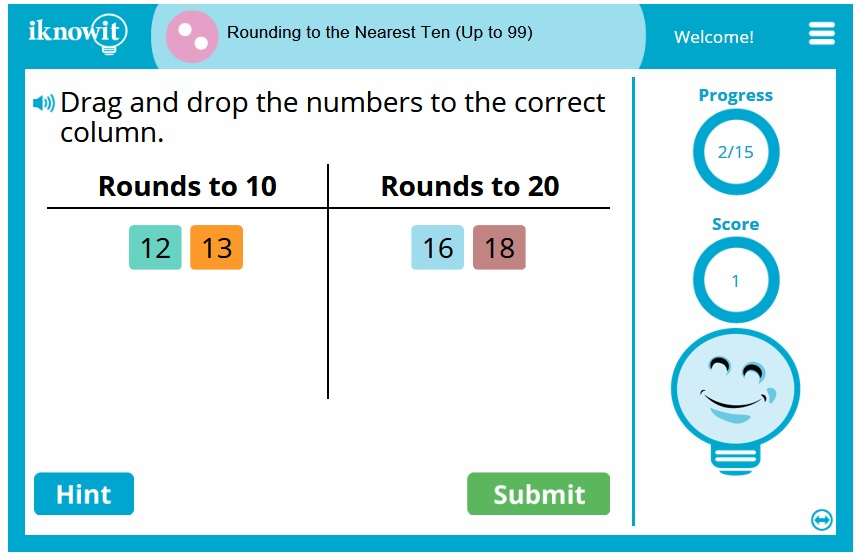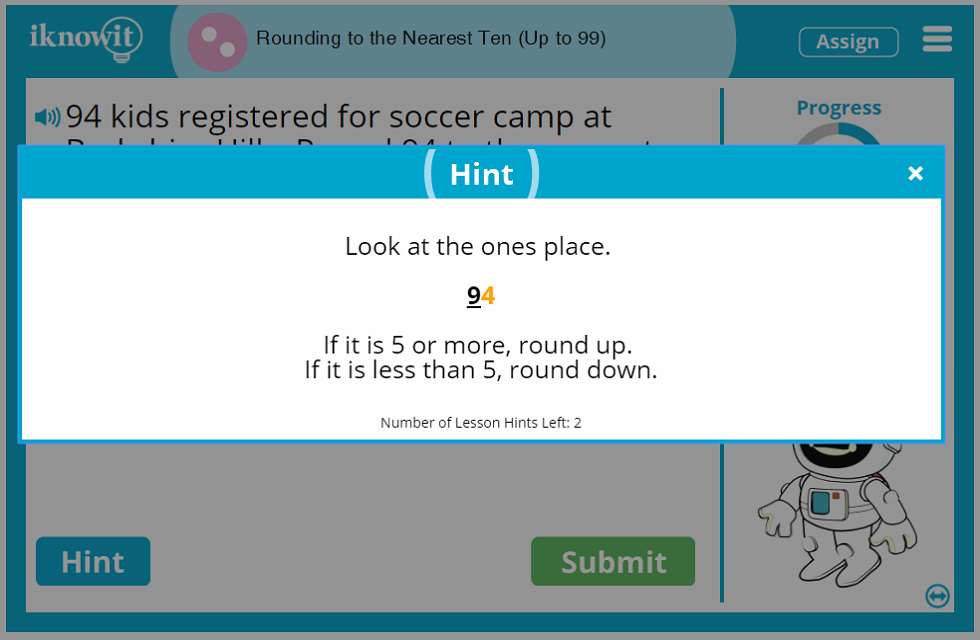## Free Lesson Preview versus Website Membership

You have the opportunity to try out "I Know It" before you purchase a membership. You can preview any lesson for free; however, the number of questions you have access to is limited until you become a member of the website.

Did you know that "I Know It" also features administrative tools for teachers? That's right! Teachers can create student logins, view their students' scores for completed lessons, adjust lesson settings, and access student progress reports.

Teachers also have flexibility in assigning lessons based on the the individual needs and skill level of their students. That's because grade levels are alternately referred to as "Level A," "Level B," "Level C," instead of "1st Grade," "2nd Grade," "3rd Grade," etc. Students won't know the lesson they're working on is associated with a specific grade level, and teachers can make sure the skill is suited to the child's ability.

## Students Love iKnowIt.com!

We are eager for you and your students to try out this dynamic math activity in your rounding lesson. Will you give it a try today? Rounding to the nearest ten is amazingly fun on "I Know It"! While you're at it, remember to sample more of our second grade math games.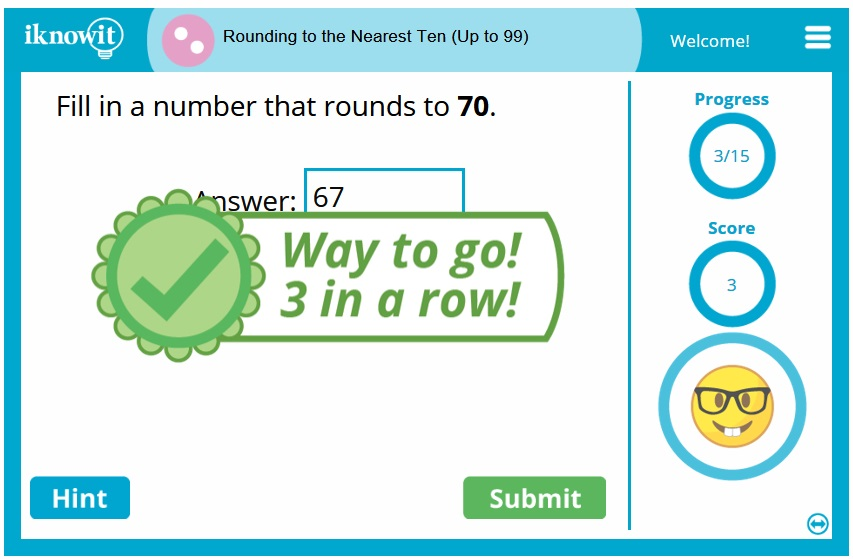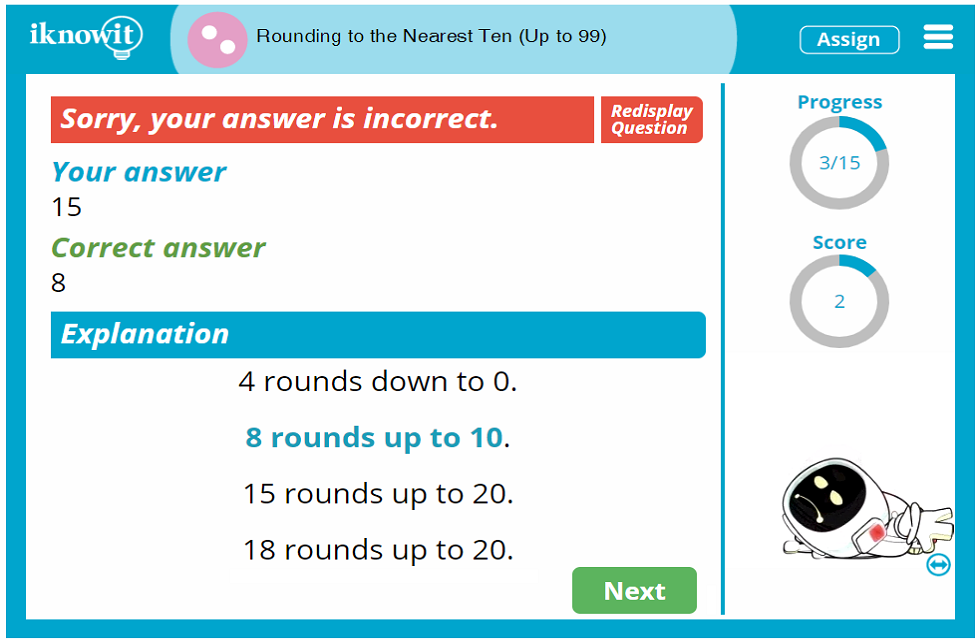## Level

We've categorized this math activity for rounding to the nearest ten as a Level B lesson. It may be appropriate to use at a first grade, second grade, or third grade level.

## Common Core Alignment

2.NBT.4
Number and Operations in Base Ten: Understand Place Value

Students should be able to compare two three-digit numbers based on their understanding of the hundreds, tens, and ones digits, using the symbols >, <, and = to distinguish greater than, less than, and equal to comparisons.

## You might also be interested in...

Rounding to the Nearest Ten (2 and 3-Digit Numbers) (Level C)
In this math activity, students will practice rounding two-digit and three-digit numbers to the nearest ten.

Rounding to the Nearest Ten and Hundred (Level D)
In this interactive math lesson, students will round four-digit numbers to the nearest ten or hundred.